JOURNAL OFAPPLIEDCRYSTALLOGRAPHY
ISSN: 1600-5767
Volume 37| Part 3| June 2004| Pages 508-509

## Scattering from capped cylinders. Addendum

aDepartment of Chemical Engineering, University of Amsterdam, Nieuwe Achtergracht 166, 1018 WV Amsterdam, The Netherlands
*Correspondence e-mail: kaya@science.uva.nl

(Received 5 January 2004; accepted 10 March 2004)

In a recent paper [Kaya (2004). J. Appl. Cryst. 37, 223–230], the scattering function and the radius of gyration of a cylinder with globular end-caps (a barbell) were presented. It was shown that the model covered several geometries by simply varying three variables. In this addendum, it is pointed out that the model, without any changes, contains the scattering functions of two other distinct shapes, namely a cylinder with small end-caps, which in the no-cylinder limit becomes a lens-shaped disc. The scattering function of a regular cylinder with flat ends (no end-caps) is also included in the model.

In a preceding paper (Kaya, 2004), we presented expressions for the scattering function and radius of gyration of a cylinder with globular end-caps. The deduction of this model was motivated by rod-like micelles, for which experiments (Bernheim-Groswasser et al., 2000) and theory (May & Ben-Shaul, 2001) suggest that the radii of the end-caps are greater than the radius of the cylindrical mid section, as illustrated in Fig. 1. By setting the length of the middle cylinder to L = 0, one obtains the scattering function of a dumbbell, a geometry of importance in colloidal (van Blaaderen & Vrij, 1992) and biological (Shi et al., 1996) systems. The barbell form factor represents an extension of the spherocylinder form factor deduced by Cusack (1981), where the radius of the spheres defining the end-caps, R, equals that of the cylinder, r. In the original paper (Kaya, 2004), the end-cap radius R was constrained to be larger than or equal to r, so that the end-caps appeared as hemispheres or bulbs appended to the flat ends of the cylinder, as shown in Fig. 1. The scattering amplitude is related to the directly measured form factor by P(Q) =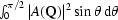, where the integration represents the averaging over all orientations of the barbell, given by the anglebetween the vector Q and the long axis of the barbell. The scattering amplitude of a barbell was found to be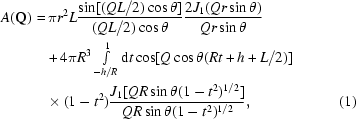where r is the cylinder radius, L the cylinder length and R (≥r) the radius of the end-cap segments. The auxiliary variable h is given by (R2r2)1/2 and gives the distance from the centre of the circle defining the end-cap to the cylinder end, as illustrated in Fig. 1. The natural progression, however, is to consider smaller end-caps, which would appear as semi-lenses attached to the cylinder ends. In this case, the zero-cylinder case (L = 0) would give the scattering amplitude of circular disks with a lens-shaped cross section, shown in Fig. 2(b). A close inspection of equation (1)actually reveals that the only modification necessary to include these small end-caps is to allow h to take negative values. In this case the absolute value of h [= −(R2r2)1/2] still gives the distance between the cylinder end and the centre of the circle defining the end-cap, with the difference that the centre is now located inside the cylinder, as illustrated in Fig. 2(a). Summarizing, one now has Rh ≥ −R. The lower limit (h = −R) applies when Rr, in which case the end-cap contribution to A(Q) disappears, leaving the scattering amplitude of a regular cylinder with radius r and length L. In the opposite limit, h = R (r = 0), the scattering amplitude is that of two full spheres whose surfaces are separated by a distance L.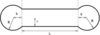Figure 1 The model of the barbell with the relevant geometrical parameters.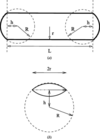Figure 2 (a) Model of the capped cylinder (thick solid line). The dashed circles define the radius of curvature R of the end-caps. (b) Cross section of a circular lens (thick solid line) with radius r and maximum thickness 2(R − h).

Scattering form factors of circular lenses with different curvatures are shown in Fig. 3. For R = r (h = 0) one recognizes the characteristic oscillations of the sphere form factor. The form factors corresponding to thick lenses follow a Q−4 Porod law at intermediate and high Q, with very weak oscillations. The overall flat structure of the thinnest lenses produces the Q−2 regime at intermediate Q values.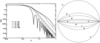Figure 3 Left: normalized scattering form factors of circular lenses with radius r = 100 and varying radii of curvature R. The flattest geometries (R = 500 and R = 1000) exhibit a Q−2 regime around Q = 0.1, a sign of their overall two-dimensional structure. Right: cross sections of the circular lenses: a, full sphere of radius R = r = 100; b, R = 150; c, R = 200; d, R = 500; e, R = 1000.

In the main paper, the radius of gyration of the barbell, Rg, was also presented. Since the equations for end-cap volume and curved surface area given in the main paper (Kaya, 2004) are valid also for the small caps (with h < 0), we let the expression for Rg cover all the geometries in question, by explicitly writing the contributions from the cylindrical part as r-dependent terms, and the terms arising from the end-caps as functions of the variables R and h. The radius of gyration then reads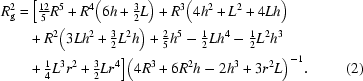With h = −R, all of the R-dependent terms cancel out, leaving Rg2 for a regular cylinder of radius r and length L.

In conclusion, we have demonstrated that the scattering-amplitude equation (1), originally devised for barbells and dumbbells, encompasses additional scattering geometries. The most important are the circular lens and the capped cylinder. Equation (1)thus contains the scattering functions of seven distinct geometries: cylinder, capped cylinder, barbell, spherocylinder, sphere, dumbbell and lens. This makes it a versatile basis for modelling the scattering curves of several systems. With regard to such experimental applications, the circular lens scattering function should provide a good model for scattering from, for instance, liquid droplets embedded in a gel (Walsh et al., 1996), self-assembled quantum dots (Sauvage et al., 20002), and precipitates or cavities in ceramic materials (Page et al., 1984).

### ReferencesJOURNAL OFAPPLIEDCRYSTALLOGRAPHY